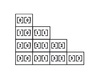Array

• ## C语言中数组的总结

万次阅读 多人点赞 2018-05-26 13:43:41
目录 一维数组的创建和初始化 一维数组的使用 一维数组在内存中的存储 ...数组的创建：在创建数组时，我们必须定义数组的类型和大小，数组的大小不能为0，数组中的元素类型都是相同的。 eg: int arr
#目录 #

一维数组的创建和初始化
一维数组的使用
一维数组在内存中的存储
指针的初步介绍
一维数组的指针访问
二维数组的创建和初始化
二维数组的使用
二维数组在内存中的存储
二维数组的指针访问
有关数组的运算
数组作为函数参数

1.一维数组的创建和初始化
数组的创建：在创建数组时，我们必须定义数组的类型和大小，数组的大小不能为0，数组中的元素类型都是相同的。
eg:
int arr;//[]内必须是常量/常量表达式(3+8)，不能是一个变量(x...)

数组的初始化：在数组创建时，我们也要必须为数组初始化。
eg:
int arr1 = {1, 2, 3};
int arr2[] = {1, 2, 3};//在这里，我们arr里边的数字可以不用写；
int arr3 = {1, 2};//也是可以的，只是把最后一个数初始化为0了而已
int arr4 = {1, 2, 3, 4};//是不可以的，不能超过数组长度
char arr5 = {'a', 98, 'c'};//因为是字符类型，所以98其实就是字符'b'
char arr6[] = "abcdef";

#include<stdio.h>

int main()
{
char arr1[] = { 'a', 'b', 'c' };
char arr2 = "abc";
char *p = "abc";//这里只是把a的地址放进了p里边

return 0;
}

在内存中的存储：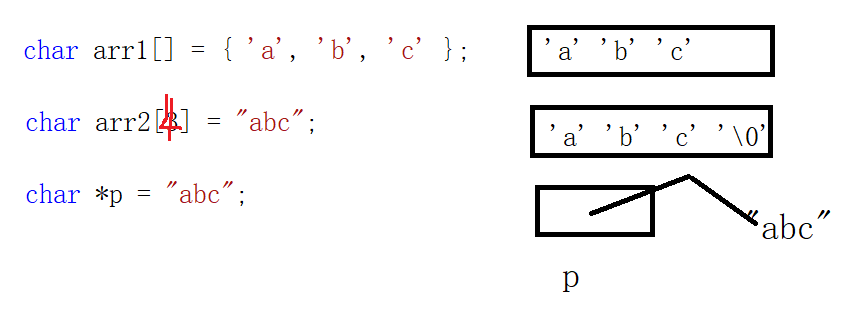2.一维数组的使用
eg:
#include<stdio.h>

int main()
{
int arr = { 0 };
int i = 0;
for (i = 0; i < 10; i++)//i<11是不可以的，不可以越界访问
{
arr[i] = i;
}

return 0;
}

数组是使用下标来访问的，下标是从0开始。
数组的大小可以通过计算得到。(sz = sizeof(arr)/sizeof(arr));
3.一维数组在内存中的存储
eg:
#include<stdio.h>

int main()
{
int arr = { 0 };
int i = 0;
for (i = 0; i < sizeof(arr)/sizeof(arr); i++)
{
printf("&arr[%d] = %p\n", i, &arr[i]);
}

return 0;
}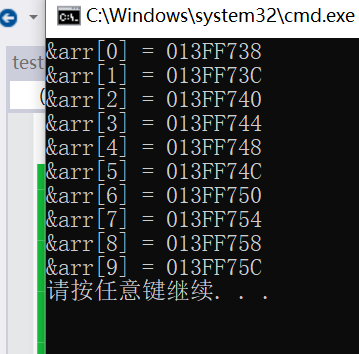从输出结果我们可以看出数组元素在内存中的存储是：地址是由低到高并且是连续存储的。
4. 指针的初步介绍
指针可以理解为一个变量，是一个专门用来存放地址的一个变量。
eg:
int *ptr = NULL;//定义一个整型的指针变量，初始化为NULL
char *ptr = NULL;//定义一个字符的指针变量，初始化为NULL

5. 一维数组的指针访问
eg:
#include<stdio.h>

int main()
{
int arr = { 0, 1, 2, 3, 4, 5, 6, 7, 8, 9 };
printf("%p\n", arr);
printf("%d\n", *arr);

return 0;
}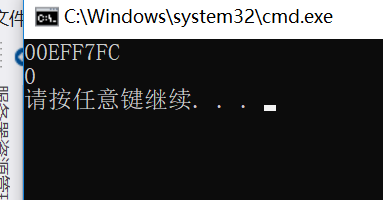从输出结果我们可以看出数组名其实存放的就是首元素的地址
eg:
int main()
{
int arr = { 0 };
int i = 0;
int sz = sizeof(arr) / sizeof(arr);
int *p = arr;
for (i = 0; i < sz; i++)
{
//arr[i] = i; (1)
*(p + i) = i; (2)
}

for (i = 0; i < sz; i++)
{
printf("%d\n", arr[i]);//(1)和(2)的输出结果一样
}

return 0;
}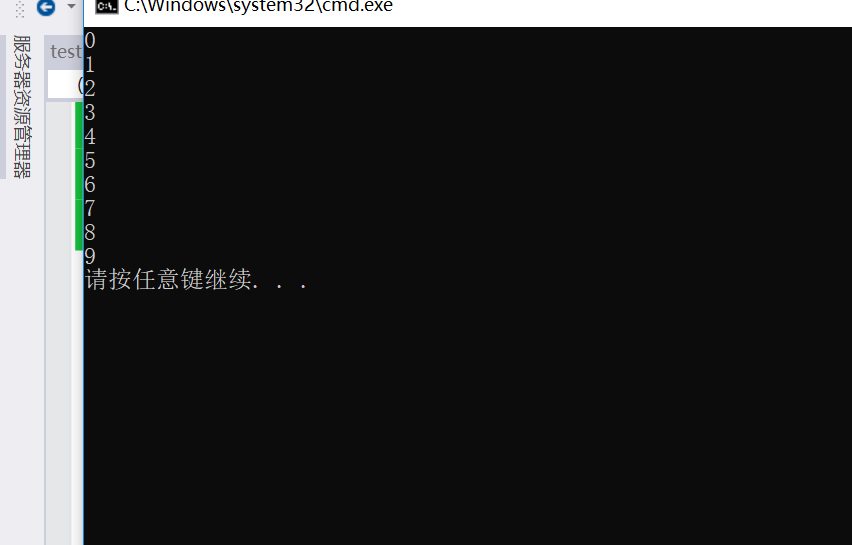从输出结果我们可以看出，我们定义了一个指针p，指向arr，然后我们通过指针来访问数组。
6. 二维数组的创建和初始化
eg:
#include<stdio.h>

int main()
{
int arr = { 1, 2, 3, 4, 5, 6 };
//int arr[] = {{1, 2},{3, 4, 5},{6}};可以
//arr[] = {{1, 2},{3, 4, 5},{6}}；是不可以的

return 0;
}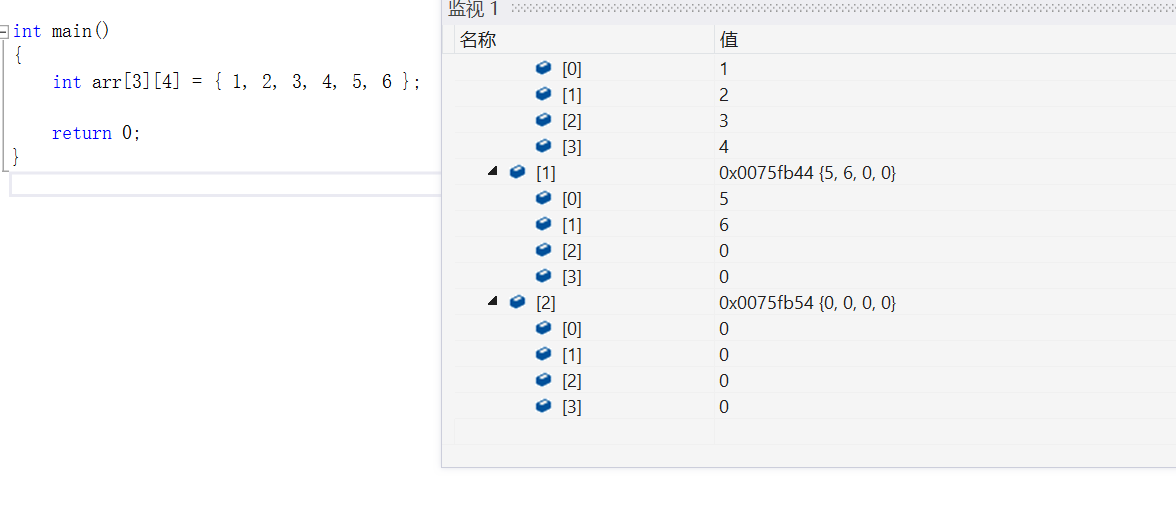从监视窗口我们可以看到，一行有四个元素，总共有三行，当后边元素没有初始化的时候，全默认为0。
7. 二维数组的使用
二维数组的使用也是通过下标方式
eg:
#include<stdio.h>

int main()
{
int arr = { 0 };
int i = 0;
int j = 0;
for (i = 0; i < 3; i++)
{
for (j = 0; j < 5; j++)
{
arr[i][j] = i * 5 + j + 1;
}
}

for (i = 0; i < 3; i++)
{
for (j = 0; j < 5; j++)
{
printf("%d ", arr[i][j]);
}
printf("\n");
}

return 0;
}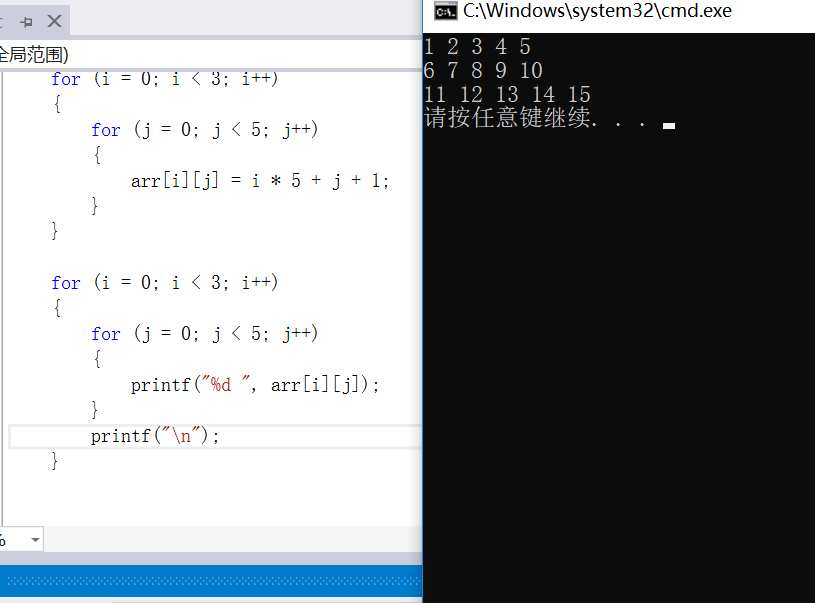8. 二维数组在内存中的存储
我们可以通过打印它的地址来观察它是如何存储的。
eg:
#include<stdio.h>

int main()
{
int arr = { 0 };
int i = 0;
int j = 0;
for (i = 0; i < 3; i++)
{
for (j = 0; j < 5; j++)
{
printf("&arr[%d][%d] = %p\n", i, j, &arr[i]);
}
}

return 0;
}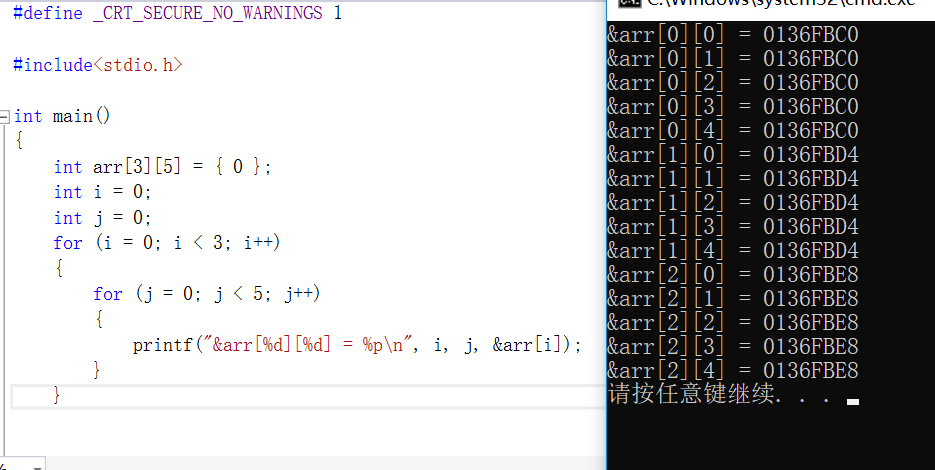我们可以看出数组元素的地址是连续的，并没有进行换行，我们只是把它假想成了三行。
9. 二维数组的指针访问
我们知道了一维数组的内存存储模式之后，我们尝试使用指针对一维数组进行访问，那在这里，我们尝试使用指针来访问我们的二维数组。
eg:

#include<stdio.h>

int main()
{
int arr = { 0 };
int *p = &arr;
int i = 0;
int j = 0;
for (i = 0; i < 15; i++)
{
*(p + i) = i + 1;
}
for (i = 0; i < 3; i++)
{
for (j = 0; j < 5; j++)
{
printf("%d ", arr[i][j]);
}
}

return 0;
}


指针也存在类型：
eg:
#include<stdio.h>

int main()
{
int num = 0x11223344;
int *p = &num;
*p = 0;

return 0;
}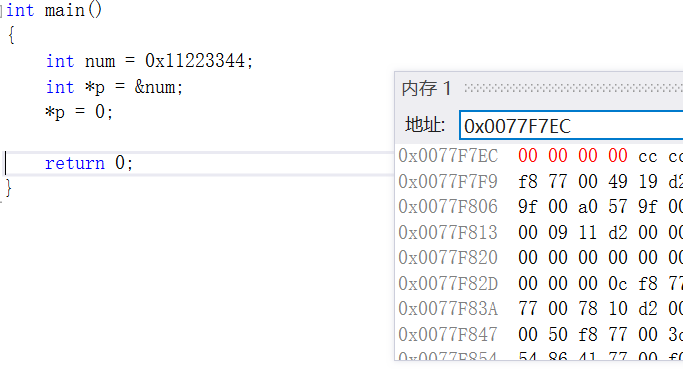这里的指针p是int型，所以我们对它的改变，是改变4个字节的大小，同样的，如果我们对p进行加1操作，指针向后跳4个字节。
eg:
#include<stdio.h>

int main()
{
int num = 0x11223344;
//int *p = &num;
//*p = 0;
char *pc = &num;
*pc = 0;

return 0;
}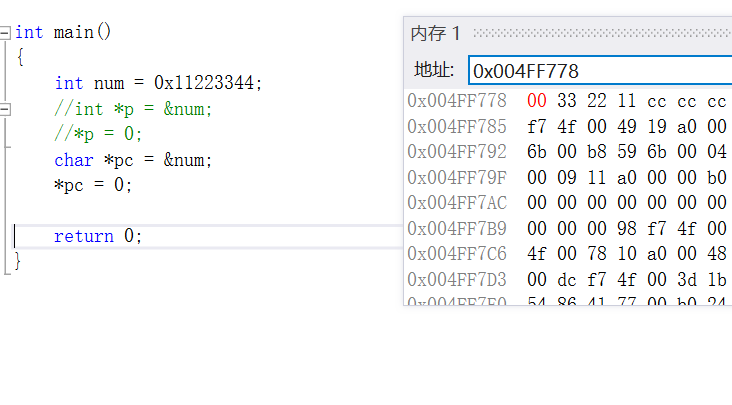我们可以从内存中观察到，只改变了1个字节的大小，因为pc指针的类型是char。如果我们对pc进行加1操作，指针向后跳1个字节。
eg:
#include<stdio.h>

int main()
{
int arr = { 0 };
printf("%p\n", &arr);
printf("%p\n", &arr + 1);
printf("---------------\n");
printf("%p\n", arr);
printf("%p\n", arr + 1);
printf("---------------\n");
printf("%p\n", &arr);
printf("%p\n", &arr + 1);

return 0;
}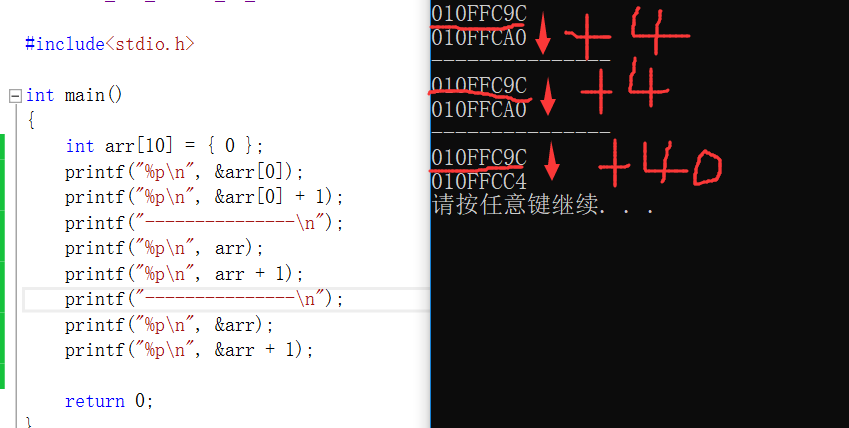我们可以从输出结果看出&arr,arr,&arr它们的地址虽然相同，但它们的意义是不同的，&arr，取的是数组的地址，对它进行加1，就相当于跳过整个数组，又因为数组是int型，总共有10个元素，所以跳过的字节大小是40。
10. 有关数组的运算##
关于数组我们必须要学会有关数组的一些运算。
eg:
#include<stdio.h>

int main()
{
//一维数组
int a[] = { 1, 2, 3, 4 };
printf("%d\n", sizeof(a));//16
//1.数组名单独放在sizeof内部，数组名表示整个数组，所以sizeof(数组名)计算的是是数组总大小，单位是字节
//2.&数组名，数组名表示整个数组，所以&数组名取出的是整个数组的地址
//3.除此之外，所有的数组名都表示首元素的地址
printf("%d\n", sizeof(a + 0));//4    a代表首元素地址，a+i代表第i个元素的地址，在32位平台下所有的地址的大小都是4个字节
printf("%d\n", sizeof(*a));//4       a是首元素地址，*a是首元素--1，int型占4个字节大小
printf("%d\n", sizeof(a + 1));//4    a是首元素地址，a+1是第二个元素的地址，它还是一个地址
printf("%d\n", sizeof(a));//4     a--第二个元素
printf("%d\n", sizeof(&a));//4       &a虽然取出的是整个数组的地址，但它还是一个地址
printf("%d\n", sizeof(*&a));//16     &a取出的是整个数组的地址，对它进行解引用，就是这个数组，这个数字的大小就是16
printf("%d\n", sizeof(&a + 1));//4   &a取出的是整个数组的地址，加1跳过了整个数组（16个字节），但它还是一个地址
printf("%d\n", sizeof(&a));//4    &a取的是第一个元素的地址
printf("%d\n", sizeof(&a + 1));//4   &a + 1取的是第二个元素的地址

return 0;
}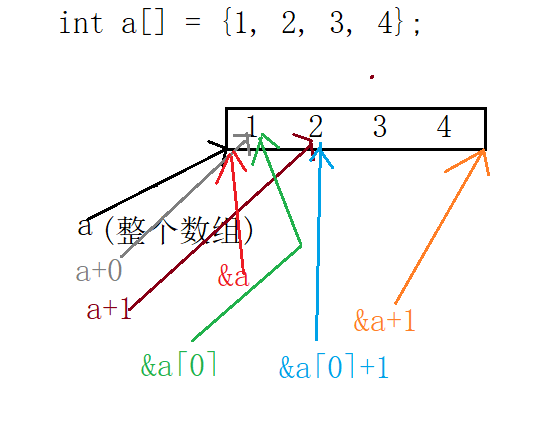#include<stdio.h>

int main()
{
//字符数组
char arr[] = { 'a', 'b', 'c', 'd', 'e', 'f' };
printf("%d\n", sizeof(arr));//6
printf("%d\n", sizeof(arr + 0));//4        首元素地址
printf("%d\n", sizeof(*arr));//1           首元素地址解引用是首元素（a），char类型占1个字节
printf("%d\n", sizeof(arr));//1         首元素
printf("%d\n", sizeof(&arr));//4           数组的地址
printf("%d\n", sizeof(&arr + 1));//4       下一个数组的地址，跳过了f
printf("%d\n", sizeof(&arr + 1));//4    第二个元素的地址

printf("%d\n", strlen(arr));//随机值       strlen()求的是字符串长度，以'\0'为结束标志，这里并没有'\0'，所以会一直往后数
printf("%d\n", strlen(arr + 0));//随机值   还是从'a'开始数，但没有'\0'，所以停不下来
printf("%d\n", strlen(*arr));//程序会崩掉  strlen()接收的是一个地址，*arr是字符'a'，这里把'a'的ASCII码值（97）作为一个地址访问，这一块的地址是不能被访问的
printf("%d\n", strlen(arr));//错误      传的是'b'，和传的是'a'效果一样
printf("%d\n", strlen(&arr));//随机值      &arr虽然取的是数组的地址，但数组的地址和数组首元素的地址是一样的，也是从‘a'开始数，但并没有'\0'
printf("%d\n", strlen(&arr + 1));//随机值  但这个随机值和前边的随机值意义不同，它是把'a','b','c','d','e','f'跳过去了，从f后边开始数
printf("%d\n", strlen(&arr +1));//随机值   这个是从'b'开始往后数的

return 0;
}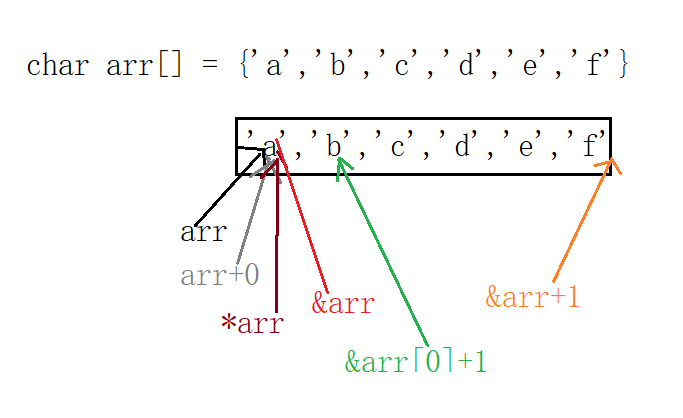#include<stdio.h>

int main()
{
char arr[] = "abcdef";
printf("%d\n", sizeof(arr));//7   里边还有'\0'，只不过我们看不到而已
printf("%d\n", sizeof(arr + 0));//4     arr+0---首元素地址
printf("%d\n", sizeof(*arr));//1   对首元素地址解引用是首元素
printf("%d\n", sizeof(arr));//1 第二个元素
printf("%d\n", sizeof(&arr));//4    数组的地址也是地址
printf("%d\n", sizeof(&arr + 1));//4  也是一个地址，不过这个地址在'\0'后边，跳过了整个数组
printf("%d\n", sizeof(&arr + 1));//4   从b开始的一个地址

printf("%d\n", strlen(arr));//6   strlen()以'\0'为结束标志，但不算'\0'
printf("%d\n", strlen(arr + 0));//6  arr+0与arr都代表首元素地址
printf("%d\n", strlen(*arr));//错误   这传进来的不是一个地址，而是一个字符
printf("%d\n", strlen(arr));//错误
printf("%d\n", strlen(&arr));//6    数组的地址也是首元素地址，地址的位置是一样的
printf("%d\n", strlen(&arr + 1));//随机值   跳过了'\0'，从'\0'往后数，不知道会数到哪里去
printf("%d\n", strlen(&arr + 1));//5    从第二个元素（b）开始往后数，遇到'\0'结束

return 0;
}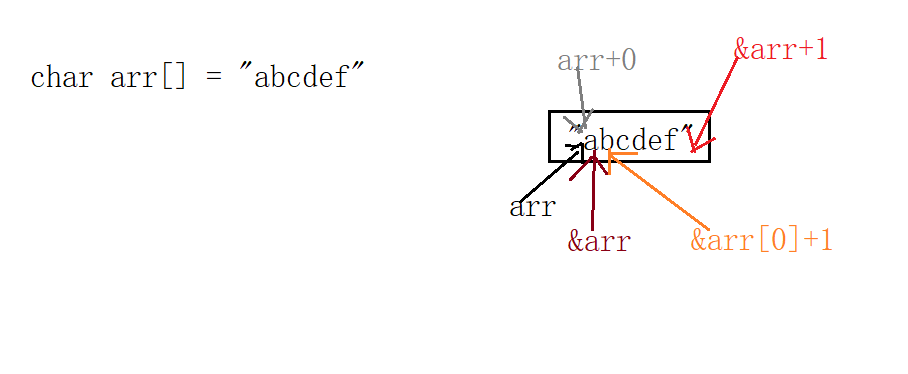#include<stdio.h>

int main()
{
char *p = "abcdef";
printf("%d\n", sizeof(p));//4   p是指针变量，里边存的是a的地址
printf("%d\n", sizeof(p + 1));//4   还是一个地址，不过是指向了b的地址
printf("%d\n", sizeof(*p));//1      对a的地址解引用就是a
printf("%d\n", sizeof(p));//1    第一个元素（a）
printf("%d\n", sizeof(&p));//4      &p取的是p的地址，p是一个指针，指向a的地址，但p的地址是什么并不知道
printf("%d\n", sizeof(&p + 1));//4  &p+1--跳过了p的一个地址
printf("%d\n", sizeof(&p + 1));//4   还是一个地址，这个地址指向了b的地址

printf("%d\n", strlen(p));//6        从a开始向后数
printf("%d\n", strlen(p + 1));//5    从b开始向后数
printf("%d\n", strlen(*p));//错误    *p就是a，strlen()要的是一个地址，而不是a的ASCII码值（97）
printf("%d\n", strlen(p));//错误
printf("%d\n", strlen(&p));//随机值
printf("%d\n", strlen(&p + 1));//随机值
printf("%d\n", strlen(&p + 1));//5    从b开始往后数

return 0;
}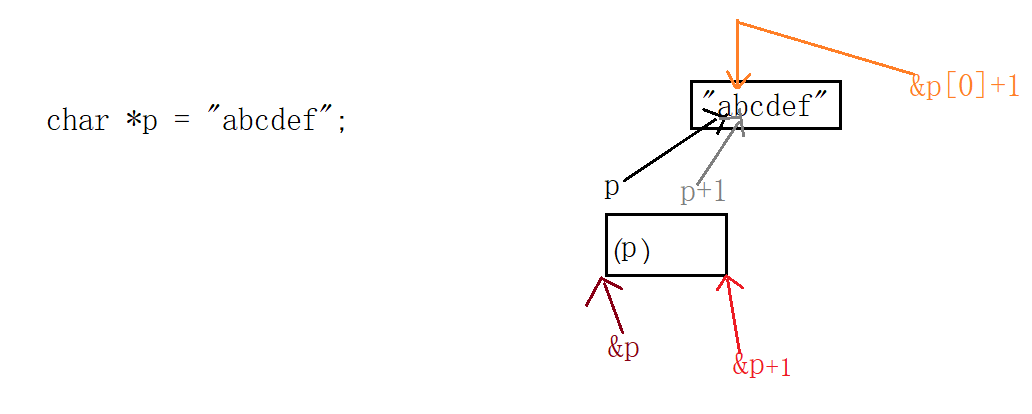#include<stdio.h>

//二维数组
int main()
{
int a = { 0 };
printf("%d\n", sizeof(a));//48    整个数组有12个元素，每个元素都是int型
printf("%d\n", sizeof(a));//4   代表的是第一行第一列那个元素
printf("%d\n", sizeof(a));//16   a--第一行数组名，第一行总共有4个元素
printf("%d\n", sizeof(a + 1));//4   a降级变为a的地址，a+1是a的地址
printf("%d\n", sizeof(a + 1));//4    a--首元素（第一行）地址，a+1--第二行地址
printf("%d\n", sizeof(&a + 1));//4    第二行地址
printf("%d\n", sizeof(*a));//16   对第一行地址解引用就是第一行元素
printf("%d\n", sizeof(a));//16    这里有好多人会出错，认为这个数组并没有这么大，只有3行，不能访问第4行，其实这里并没有访问第4行，它只是一个类型（1行的大小）
return 0;
}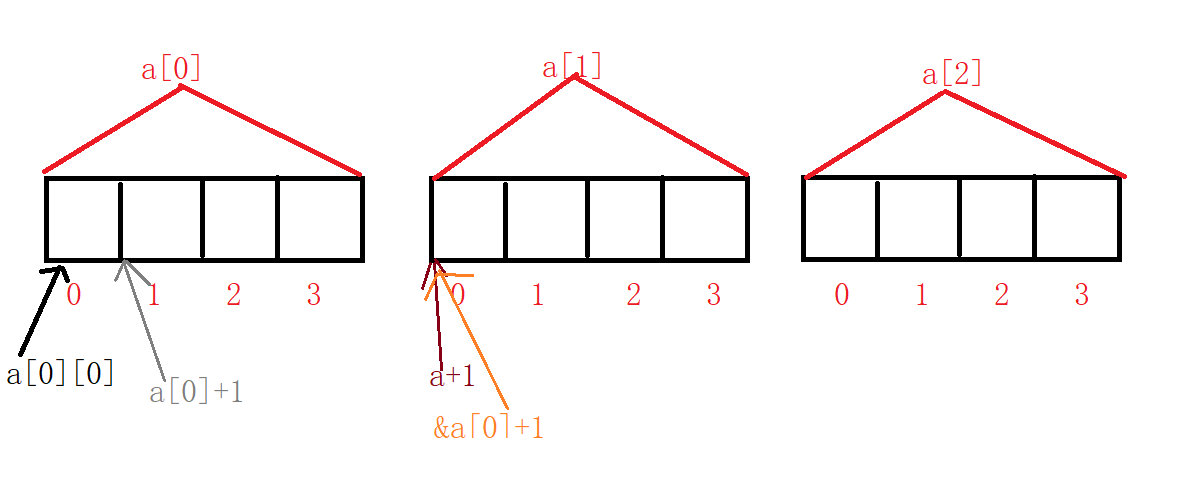11.数组作为函数参数
我们在写代码的时候，会将数组作为参数传给函数。
eg:
void bubble(int arr[])
{
int sz = sizeof(arr)/sizeof(arr);//这是错误的
...
}

数组作为函数参数时，不会把整个数组传递过去，实际上只是把数组的首元素地址传递过去了。


展开全文• ## 数组

千次阅读 多人点赞 2018-07-28 16:31:16
数组也是一种引用类型， 2、名称：数组名称不是固定的，与存放的数据的类型有关。 数组中只能存一种类型的数据  如: 存放一组int类型的数据，  数组名称 int[]    存放一组字符串数据  数组名称 String[] ...
1、概念：一组相同类型的数据的组合
数组也是一种引用类型，
2、名称：数组名称不是固定的，与存放的数据的类型有关。

数组中只能存一种类型的数据
如:  存放一组int类型的数据，
数组名称   int[]

存放一组字符串数据
数组名称   String[]

存放一组Scanner类型的数据
数组名称   Scanner[]

存放一组字符类型的数据
数组名称   char[]
3、元素的概念：
数组中的每个数据，都是这个数值的元素。
4、数组的声明与初始化
声明：元素类型[]  变量名;
元素类型   变量名[];--不建议此写法
变量的初始化的时机：2种
(1)声明时直接初始化
(2)先声明，再初始化(使用前初始化)
数组的变量初始化：3种情况
(1)静态初始化
String[] names = {"A","B"};
int[] nums = {};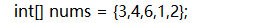(2)动态初始化
--规定数组长度的写法
int[] ages = new int[length];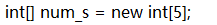其实有默认元素：
整数类型：默认是0
浮点数类型：默认0.0
字符类型：默认'\u0000'
布尔类型：默认false
引用数据类型：默认值null

--不规定长度的写法
int[] ages = new int[]{};
double[] counts = new double[]{1.2,3.14};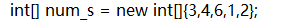5、数组的长度：
即数组元素的个数
表示方式：变量名.length;
6、数组的下标(index):
下标从0开始，最后一个元素的下标为：length-1;
下标范围：0~length-1
7、数组元素的访问(查看):
通过下标进行查看
语法:变量名[index];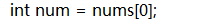元素的遍历(从头到尾依次查看)
for(int i=0;i<变量名.length;i++){
System.out.println(变量命[i]);
}
8、数组元素的覆盖(赋值，替换)

语法：变量名[index] = value;

9、数组间的赋值

数组间的变量赋值：
通过一个变量对数组的元素进行修改，
那么与之相关的变量对应的数组的元素
也会发生变化。
如：
int[] a = {1,2,3};
int[] b = a;
通过b修改元素，a对应的数组也跟着改变

数组是引用数据类型，在变量中村的是地址。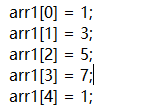10、数组下标越界异常：
java.lang.ArrayIndexOutOfBoundsException

出现的原因：
访问数组元素时的下标超出了范围[0~length-1]，属于运行时异常，

数组下标越界异常，编译器检查不出来此异常
运行时才会出现，出现的结果造成了程序的中断结束。
正确范围：0~length-1
错误下标：
变量名[-1]
变量名[length]
11、引用类型之Arrays
是数组的工具类。此类提供了很多对数组操作的功能方法
如：

在使用工具类时，一定要先到包。不然会出现错误
String info = Arrays.toString(数组名);
Arrays.toString():此方法是将数组的元素
一一拼接成字符串.(一定要是数组)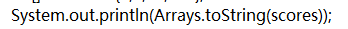格式如下：
"[元素1,元素2,.....元素length-1]"

12、数组的复制
数组的特点：一经创建，长度不可变。

系统复制法：
System.arraycopy(src,start1,des,start2,length);
src:源数组，即被复制的数组名
start1:源数组复制的开始下标
des:目标数组，即复制到的数组名
start2:目标数组复制的开始下标
length:复制的长度

int[] nums = {99,100,98,99,100};
int[] num1 = new int[nums.length];//{0,0,0,0,0}
System.arraycopy(nums,0,num1,0,nums.length);
System.out.println("num1:"+Arrays.toString(num1));

工具类提供的复制方法:
元素类型[] 变量名=Arrays.copyOf(src,newLength);
src:源数组
newLength:新数组的长度

功能解释：从src的下标为0的元素开始复制
复制到长度为newLength的新数组中
新数组存放元素的开始位置为0

（可以对复制的数组进行扩容何人缩容）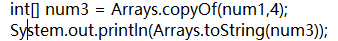数组的练习：

/* 定义一个字符串数组names,
存储10个学生的姓名*/
String[] names = new String;
for(int i=0;i<names.length;i++){
names[i] = "小"+(char)(i+65);
}
for(int i=0;i<names.length;i++){
System.out.print(names[i]+" ");
}
/* 定义一个int数组scores,用于存储这10个学生的
成绩，成绩使用随机数 [90,100]
计算成绩的平均值
找到成绩的最大值，并输出其姓名
找到成绩的最小值，并输出其姓名
*/
System.out.println();
int[] scores = new int[names.length];
for(int i=0;i<scores.length;i++){
scores[i] = (int)(Math.random()*11+90);
}
for(int i=0;i<scores.length;i++){
System.out.print(scores[i]+" ");
}
/* 计算平均值：*/
int sum = 0;
for(int i=0;i<scores.length;i++){
sum+=scores[i];
}
double avg_score = sum*1.0/scores.length;
System.out.println("平均成绩为："+avg_score);
/* 找最大值 */
int max = -1;//用于存入最大值
int min = 101;//用于存储最小值
for(int i=0;i<scores.length;i++){
if(max<scores[i]){
max = scores[i];//将较大值的成绩存入max
}
if(min>scores[i]){
min = scores[i];//将较小值的成绩存入min
}
}
System.out.println("最高成绩："+max);
System.out.println("最低成绩："+min);

/*
需要知道最高成绩的下标，才能知道人名
而最高成绩的下标可能有多个。需要保存
到一个新数组里

最低成绩同上。
*/
int h_count = 0;
int l_count = 0;
for(int i=0;i<scores.length;i++){
if(max==scores[i]){
h_count++;//统计最高成绩的个数
}
if(min==scores[i]){
l_count++;//统计最低成绩的个数
}
}
/*
将最高/最低成绩的下标：
存入新数组中
*/

int[] max_indexs = new int[h_count];//用来存储最高成绩的多个下标
int max_count=0;//用来下标的自增

int[] min_indexs = new int[l_count];//用来存储最低成绩的多个下标
int min_count=0;//用来下标的自增

for(int i=0;i<scores.length;i++){
if(scores[i]==max){
max_indexs[max_count] = i;//找到最大值的下标，并存入新数组
max_count++;//为下一次存储做准备
}
if(scores[i]==min){
min_indexs[min_count++] = i;
}
}
/*输出 最高成绩的姓名和分数
从存储下标的数组中，取出值，此值是
names数组的下标

*/
for(int i=0;i<max_indexs.length;i++){
int v = max_indexs[i];
System.out.println(names[v]+":"+scores[v]);
}

/*输出 最低成绩的姓名和分数*/
for(int i=0;i<min_indexs.length;i++){
int v = min_indexs[i];//
System.out.println(names[v]+":"+min);
}

}

二维数组：

概念：数组的元素依然是数组
int[] a = {1,2,3,4}
int[][] b={{1,2},{2,3,4,5,6},{1,2,3}}
访问： b-->{1,2}
b-->{2,3,4,5,6}
b-->{1,2,3}

b -->1
b -->2
.....
b -->5
b -->6
...
b -->2
x={
{1,2,3},
{1,2,3},
{4,5,6}
}

x数组的元素：都是等长的数组，类似于矩阵。

y ={
{1,2},
{1,2,3},
{1}
}
y数组的元素：不是等长的数组。

二维数组的初始化：
静态初始化
如：int[][] a = {{1},{1,2},{2,3,4}};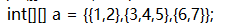动态初始化：
(1)等长式初始化
int[][] a = new int;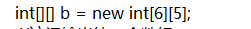表示a有三个元素，每个元素都是2个长度的数组。
(2)只规定高维数组的长度
int[][] a = new int[];
表示a有三个元素，但是都是null.
null在使用时，需要新建数组对象(长度任意)

int[][] c = new int[];
//输出c的第一个元素
System.out.println(c);//null  数组只有长度没有元素{}
c = new int;//将null替换成数组对象，如果是以上初始化方法，一定要有这一步
System.out.println(Arrays.toString(c));
c = 1;
c = 2;
System.out.println(c);
System.out.println(Arrays.toString(c));

二维数组的练习

/*录入5个学生的数学，语文，英语三科成绩
计算每科的平均成绩与每人的平均成绩
并找出每科成绩的最高分与最低分。
*/

//以学生为主体，录入每个学生的3科，此处也可以以科目为主题，录入每个学生，

//数组定义变为 int
int[][] scores = new int;
Scanner sc = new Scanner(System.in);
for(int i=0;i<scores.length;i++){

//分别录入每个学生三科成绩
System.out.println("录入第"+(i+1)+"个学生的成绩");
for(int j=0;j<scores[i].length;j++){
scores[i][j]=sc.nextInt();
}
}
/*查看 每个学生的成绩*/
for(int i=0;i<scores.length;i++){
System.out.println("学生"+(i+1)+":"+Arrays.toString(scores[i]));
}
/*计算每个学生的总成绩和平均成绩
int sum = 0;
sum+=scores;
sum+=scores;
sum+=scores;
sum/3.0;

int sum = 0;
sum+=scores;
sum+=scores;
sum+=scores;
sum/3.0;

.....
*/
for(int i=0;i<scores.length;i++){
int sum = 0;
for(int j=0;j<scores[i].length;j++){
sum+=scores[i][j];
}
System.out.println("学生"+(i+1)+"的总成绩："+sum+",平均成绩："+(int)((sum/3.0)*100)/100.0);
}
/*
计算每科的总成绩和平均成绩
int total_sum = 0;
total_sum+=scores;
total_sum+=scores;
total_sum+=scores;
total_sum+=scores;
total_sum+=scores;
total_sum+=scores;
.....
total_sum/10.0

int total_sum = 0;
total_sum+=scores;
total_sum+=scores;
total_sum+=scores;
total_sum+=scores;
total_sum+=scores;
total_sum+=scores;
.....
total_sum/10.0

int total_sum = 0;
total_sum+=scores;
total_sum+=scores;
total_sum+=scores;
total_sum+=scores;
total_sum+=scores;
total_sum+=scores;
.....
total_sum/10.0

*/
/*
顺便统计每科的最高分与最低分
*/
for(int i=0;i<scores.length;i++){
int total_sum = 0;
int max = -1;
int min = 101;
for(int j=0;j<scores.length;j++){
total_sum+=scores[j][i];

if(max<scores[j][i]){
max = scores[j][i];
}
if(min>scores[j][i]){
min = scores[j][i];
}
}
System.out.println("学科"+(i+1)+"的总成绩："+total_sum+",平均成绩："+total_sum/10.0+",最高分："+max+",最低分："+min);
}
}

引用类型数组：
基本数据类型数组，元素是基本类型的数据
引用数据类型数组，元素是对象
初始化的方式
静态初始化：
元素类型[] 变量名 = {}
动态初始化
规定长度
元素类型[] 变量名 = new 元素类型[]
不规定长度：
元素类型[] 变量名 = new 元素类型[]{}
引用数据类型的数组使用规定长度的方式进行初始化
默认值是null；存入元素时，需要新建对象存入；
如：
Cell[] cs = new Cell;
cs里有地址，数组对象的地址，此对象里有10个null
第一个元素存储（0，3）的方格
cs= new Cell(0,3)
第一个元素存储（0，4）的方格
cs= new Cell(0,4)
引用类型的数组内，存储的是元素对象的地址信息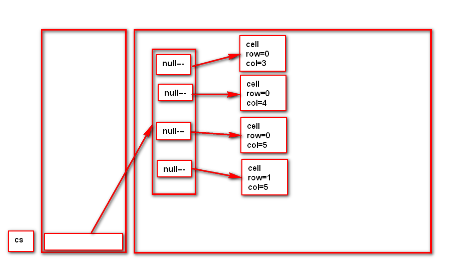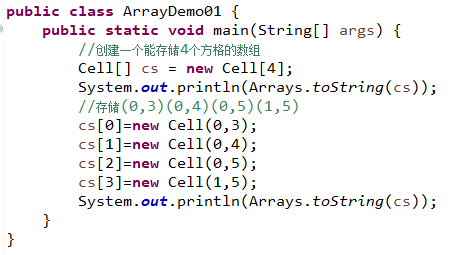展开全文• ## Java中数组的定义与使用(一)

万次阅读 多人点赞 2018-09-01 00:05:13
如果说现在要求你定义100个整型变量，那么如果按照之前的做法，可能现在定义的的结构如下： int i1, i2, i3, ... i100; 但是这儿时候如果按照此类方式定义就会非常麻烦，...
数组的基本概念
如果说现在要求你定义100个整型变量，那么如果按照之前的做法，可能现在定义的的结构如下：
int i1, i2, i3, ... i100;

但是这个时候如果按照此类方式定义就会非常麻烦，因为这些变量彼此之间没有任何的关联，也就是说如果现在突然再有一个要求，要求你输出这100个变量的内容，意味着你要编写System.out.println()语句100次。
其实所谓的数组指的就是一组相关类型的变量集合，并且这些变量可以按照统一的方式进行操作。数组本身属于引用数据类型，那么既然是引用数据类型，这里面实际又会牵扯到内存分配，而数组的定义语法有如下两类。

数组动态初始化：

声明并开辟数组：

数据类型 [] 数组名称 =  new 数据类型[长度];
数据类型 [] 数组名称 =  new 数据类型[长度];

分布进行数组空间开辟（实例化）
| Tables | Are |
| ------------- |:-------------?
| 声明数组： | 数组类型 数组名称[] = null; | | | 数组类型 [] 数组名称 =null; | | 开辟数组空间： | 数组名称 =new 数组类型[长度]; |

那么当数组开辟空间之后，就可以采用如下的方式的操作：

数组的访问通过索引完成，即：“数组名称[索引]”，但是需要注意的是，数组的索引从0开始，所以索引的范围就是0 ~ 数组长度-1，例如开辟了3个空间的数组，所以可以使用的索引是：0,1,2，如果此时访问的时候超过了数组的索引范围，会产生java.lang.ArrayIndexOutOfBoundsException 异常信息；
当我们数组采用动态初始化开辟空间后，数组里面的每一个元素都是该数组对应数据类型的默认值；
数组本身是一个有序的集合操作，所以对于数组的内容操作往往会采用循环的模式完成，数组是一个有限的数据集合，所以应该使用 for 循环。
在 Java 中提供有一种动态取得数组长度的方式：数组名称.length；

范例： 定义一个int型数组
public class ArrayDemo {
public static void main(String args[]) {
int data[] = new int; /*开辟了一个长度为3的数组*/
data = 10; // 第一个元素
data = 20; // 第二个元素
data = 30; // 第三个元素
for(int x = 0; x < data.length; x++) {
System.out.println(data[x]); //通过循环控制索引
}
}
}

数组本身除了声明并开辟空间之外还有另外一种开辟模式。
范例： 采用分步的模式开辟数组空间
public class ArrayDemo {
public static void main(String args[]) {
int data[] = null;
data = new int; /*开辟了一个长度为3的数组*/
data = 10; // 第一个元素
data = 20; // 第二个元素
data = 30; // 第三个元素
for(int x = 0; x < data.length; x++) {
System.out.println(data[x]); //通过循环控制索引
}
}
}

但是千万要记住，数组属于引用数据类型，所以在数组使用之前一定要开辟控件（实例化），如果使用了没有开辟空间的数组，则一定会出现 NullPointerException 异常信息：
public class ArrayDemo {
public static void main(String args[]) {
int data[] = null;
System.out.println(data[x]);
}
}

这一原则和之前讲解的对象是完全相同的。
数组在开发之中一定会使用，但是像上面的操作很少。在以后的实际开发之中，会更多的使用数组概念，而直接使用，99%情况下都只是做一个 for 循环输出。
数组引用传递
既然数组属于引用数据类型，那么也一定可以发生引用传递。在这之前首先来研究一下数组的空间开辟。
范例： 观察一道程序
public class ArrayDemo {
public static void main(String args[]) {
int data[] = null;
data = new int; //开辟一个长度为3的数组
data = 10;
data = 20;
data = 30;
}
}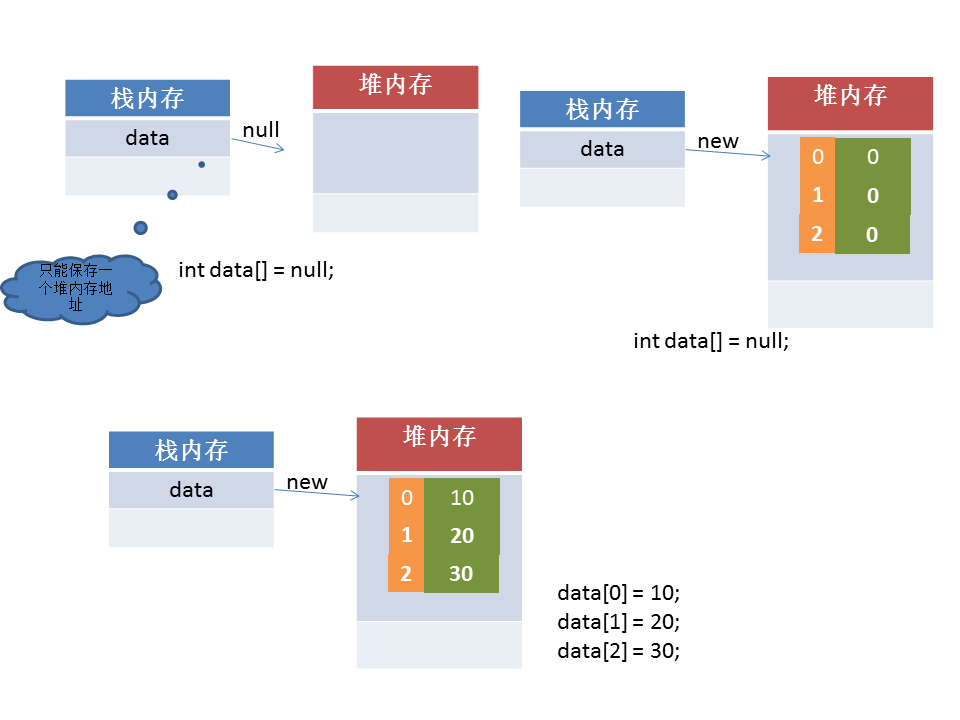那么既然说到了引用数据类型了，就一定可以发生引用传递，而现在的引用传递的本质也一定是：同一块堆内存空间可以被不同的栈内存所指向。
范例： 定义一个程序
public class ArrayDemo {
public static void main(String args[]) {
int data[] = null;
data = new int; //开辟一个长度为3的数组
int temp[] = null; //声明对象
data = 10;
data = 20;
data = 30;
temp = data;  //int temp[] = data;
temp = 99;
for(int i = 0; i < temp.length; i++) {
System.out.println(data[i]);
}
}
}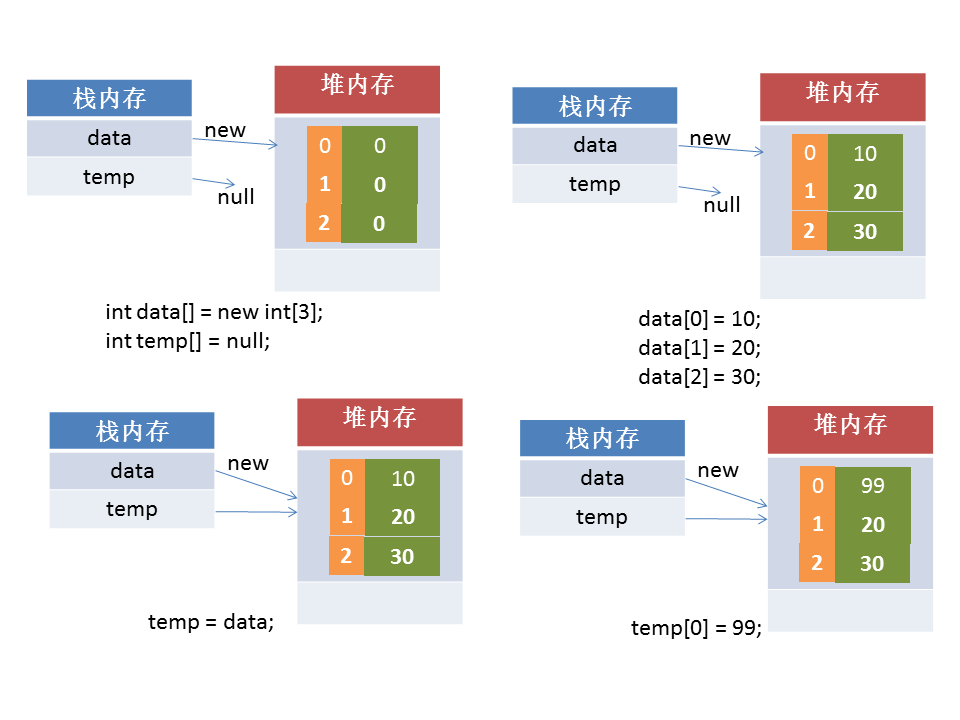引用传递分析都是一个套路。同一块堆内存被不同的栈内存所指向。
数组静态初始化
在之前所进行的数组定义都有一个明显特点：数组先开辟内存空间，而后再使用索引进行内容的设置，实际上这种做法都叫做动态初始化，而如果希望数组在定义的时候可以同时出现设置内容，那么就可以采用静态初始化完成。
数组的静态初始化一共分为以下两种类型：

Tables
Are

简化格式：
数据类型 数组名称 = {值, 值,…}

完整格式：
数据类型 数组名称 = new 数据类型[] {值, 值,…}

范例： 采用静态初始化定义数组
public class ArrayDemo {
public static void main(String args[]) {
int data[] = {1, 2, 4, 545, 11, 32, 13131, 4444};
for(int i = 0; i < data.length; i++) {
System.out.println(data[i]);
}
}
}

在开发之中，对于静态数组的初始化强烈建议使用完整语法模式，这样可以轻松地使用匿名数组这一概念。
public class ArrayDemo {
public static void main(String args[]) {
System.out.println(new int[] {1, 2, 4, 545, 11, 32, 13131, 4444}.length);
}
}

以后使用静态方式定义数组的时候一定要写上完整格式。
数组最大的缺陷：长度固定。
二维数组
在之前所使用的数组发现只需要一个索引就可以进行访问，那么这样的数组实际上非常像一个数据行的概念。

索引
0
1
2
3
4
5
6
7
8

内容
12
23
44
56
90
445
49
99
1010

现在痛过一个索引就可以取得唯一的一个记录。所以这样的数组可以简单理解为一维数组，而二维数组本质上指的是行列集合，也如果要确定某一个数据需要行索引和列索引来进行定位。

索引
0
1
2
3
4
5
6
7
8

0
12
23
44
56
90
445
49
99
1010

1
2
3
41
56
9
45
49
99
10

如果要想确定一个数据则数据使用的结构是“数组名称[行索引][列索引]”，所以这样的结构就是一个表的结构。
那么对二维数组的定义有两种声明形式：

数组的动态初始化：数据类型 对象数组[][] = new 数据类型[行个数][列个数];
数组的静态初始化：数据类型 对象数组[][] = new 数据类型[行个数][列个数]{{值, 值,…}, {值, 值,…},…};

数组的数组就是二维数组。
范例： 定义一个二维数组
public class ArrayDemo {
public static void main(String args[]) {
//此时的数组并不是一个等列数组
int data[][] = new int[][] {
{1, 2, 3}, {4, 5}, {6, 7, 8, 9}};
//如果在进行输出的时候一定要使用双重循环，
//外部的循环控制输出的行数，而内部的循环控制输出列数
for(int i = 0; i < data.length; i++) {
for(int j = 0; j < data[i].length; j++) {
System.out.print("data[" + i + "][" + j + "]=" + data[i][j] + "、");
}
System.out.println();
}
}
}

由于输出麻烦，所以可以忽略了，在进行开发之中，出现二位数组的几率并不高。


展开全文• ## 数组的三种定义方式

万次阅读 多人点赞 2017-12-25 16:16:58
1.数组的定义 用于存储同一类型数据的集合，其实数组就是一个容器。 连续的存储单元 2.数组的好处 自动给数组中的元素从零开始编号。自动给下标，从零开始0-1-2-3-…… 3.书写格式    元素类型[] 数组名 =...
1.数组的定义

用于存储同一类型数据的集合，其实数组就是一个容器。

连续的存储单元

2.数组的好处

自动给数组中的元素从零开始编号。自动给下标，从零开始0-1-2-3-……

3.书写格式

元素类型[]  数组名 =    new   元素类型[]{元素，元素，元素……};

格式1：      int [] arr = new int;

需要手动赋值arr=1;

arr=2;

arr=3

arr=4;

arr=5;

格式2：   int [] arr = new int[]{1,3,5,7｝

格式3： int[]arr = {1,3,5,7};

4.数组的内存结构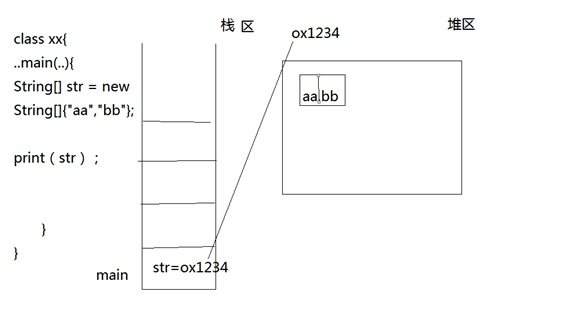5.数组的内存特点

（1）.因为使用了关键字new 会在堆内存中开辟相应的空间，分别赋予了两个数组不同的地址。当比较的时候，比较的是两个数组的地址，则为不同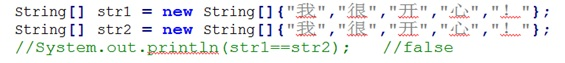（2）.本例虽未使用new 关键字，但是同样的比较的也是数组的地址；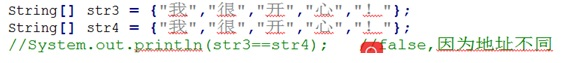（3）.此例输出为true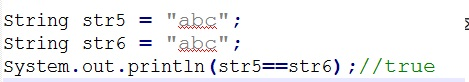因为他们指的都是同一个对象abc的地址，所以是相同的

`
展开全文• ## 数组的所有方法

千次阅读 2019-03-04 09:20:39
JavaScript中创建数组有两种方式 （一）使用 Array 构造函数： var arr1 = new Array(); //创建一个空数组 var arr2 = new Array(20); // 创建一个包含20项的数组 var arr3 = new Array(“lily”,“lucy”,“Tom”);...
• 数组 一、数组的定义 1、数组：就是用于存储相同数据类型的数据的容器 2、使用数组的原因： （1）没有数组：存在多个变量，每一个变量都需要有一个名称，并且在一个作用域内，这些名称还不能重复，如果要使用数据，...java 后端
• ## Array [] 数组

千次阅读 2018-08-12 17:55:45
※ Array 数组 数组是指一组数据的集合，（容器）数组中的每个数据称为元素。在Java中，数组也是Java对象。数组中的元素可以是任意类型(包括基本类型和引 用类),但同一个数组里只能存放类型相同的元素。长度不可变...
• 前面学习数据结构的过程中，总是使用数组作为顺序表的底层实现，给我们一种 "数据结构中，数组的作用就是实现顺序表" 的错误认识。其实，数组的作用远不止于此。 本节将从数据结构的角度讲解数组存储结构...数据结构
• ## C语言数组用法小技巧

万次阅读 多人点赞 2018-12-03 21:09:23
这可能是C语言的第四次教程，主要是讲讲数组的一些用法吧。 一.数组的下标记录用法 首先，我们看例题吧首先我们看例题： 某个超大社区需要统计社区人数，给定为n（0&lt;=n&lt;=10000）人，我们需要写一个...教程
• ## C语言数组当参数传递

万次阅读 多人点赞 2018-01-29 19:57:23
在学习C语言的过程中遇到数组作为参数传递的问题 一维数组：#include int test2(int a[]){ for(int i=0;i;i++){ printf("%d",a[i]); } } int main(){ int a = {1,2,3,4,5},*p; p = a; test2(a); } ...传值
• C语言数组部分练习题的选择题，带答案，适合初学者对数组部分的练习使用。
• 原创C语言数组形链表操作的具体接口，定义简单，逻辑清晰
• 先摆代码: ... //定义数组,不设初值 char a = { 'a' }; //定义数组,设置初值,这样其余为\0 scanf("%s", a); //第一种输入方式 int i = 0; //第二种输入方式 for (; i (a) - 1; i++) { char基础
• C语言中，数组属于构造数据结构。一个数组可以分解成多个数组元素，这些数组元素可以是基本数据类型或是构造类型。因此按照数组元素的类型不同，数组又可分为数值数组、字符数组、指针数组、结构数组等各种类别。 ...字符串 杨辉三角形
• C语言目录 C/C++学习资源（百度云盘链接） 计算机二级资料（过级专用） C语言学习路线（从入门到实战） 编写C语言程序的7个步骤和编程机制 C语言基础-第一个C程序 ...C语言数组——一维数组 二维数组 前面介绍了...
• ## c语言数组详解

万次阅读 多人点赞 2007-04-24 16:37:00
Ｃ语言中， 数组属于构造数据类型。一个数组可以分解为多个数组元素，这些数组元素可以是基本数据类型或是构造类型。因此按数组元素的类型不同，数组又可分为数值数组、字符数组、指针数组、结构数组等各种类别。...语言 c input string 存储
• ## c语言数组赋值

万次阅读 2018-02-20 13:51:33
一、数组定义原则： 长度是常量，赋值用大括号括起来。 例： int data = {5, 2, 4, 0, 3}; 如果用变量： int n=5; int a[n] = { 1,2,3,4,5 }; gcc报错： error: variable-sized object may not be ...
• ## C语言数组知识点总结

万次阅读 多人点赞 2015-03-12 18:36:10
数组：就是只能存放一种数据类型，比如int类型的数组、float类型的数组，里面存放的数据称为“元素” 数组的定义： 首先声明数组的类型，然后声明数组元素的个数（也就是需要多少存储空间） 格式： 元素类型 数组...c
• 若定义了一个数组“int a={1,2,3,4,5};”，又定义了一个数组“int b;”，那么如何编写程序才能将数组...前面说过，a 和 b 是数组名，而数组名表示的是数组“第一个元素”的“起始地址”。即 a 和 b 表示的是地...
• 这是我用c语言写的程序，我的其他资源都是免费的，是对于c语言初学者的帮助比较大的，其中有数据结构，window编程。我也在学c语言，每当我写完一个程序，我都会免费发上来。
• 将任意图片转换为C语言数组的程序，非常好用。 直接流览打开bmp或JPG图片，点convert即可转换为同名的.c文件，方便在嵌入式硬件，应用编译时，没有文件系统不能读取文件的问题。
• ## 理解c语言数组类型

千次阅读 2018-12-02 12:52:38
抛砖：数组类型，压死初学者的三座大山 1、数组类型；数组名 2、数组指针；与数组名同等级还是上一级需要&amp;才能赋值 3、数组类型和数组指针的关系； 先以一维数组为例；int a; int *a1; int （*a2）;...
• ## C语言数组+冒泡排序

千次阅读 多人点赞 2019-02-03 17:17:58冒泡排序
• C语言数组专题知识点易错点总结及做题感悟 一.知识点总结 1.一维数组的定义和初始化. 注: ① C语言数组的下标都是从0开始的; ②在定义数组时不能使用变量定义数组的大小，如果前面没有对n进行宏定义 #define n 5 则...学习总结
• 看下面代码：int main() { int a={3,6,4,2,5}; char *ptrChar="hello world";//ptrChar是char类型的指针，指向内存空间 int *ptrInt=a;//ptrInt是指向int类型的指针 printf("...指针
• ## C语言数组之排序

千次阅读 2019-03-25 19:56:34
输入某门课的成绩，按编程将分数从高到低顺序进行排序输出 第一种：采用交换法排序，也称作冒泡排序。 基本过程是先将第一个数分别于后面的数一个一个进行比较，若后面的数小，则交换后面这个数和第一个数的位置，...
• ## c语言数组元素逆序

千次阅读 2017-08-12 21:11:13
由于同一数组中的元素地址连续---建议c语言中对数组的操作，尽量用指针操作数组元素（详见方法2）---减少开销  */  #include int main() {  int arr[]={1,3,545,23}; int i=0,j=0; int n=0,temp=0; ...
• 转自：http://blog.csdn.net/xgmiao/article/details/9570825 点击打开链接数组作为函数实参：C语言数组作为函数实参时，编译器总是将其解析为指向数组首元素地址的指针(地址调用)原因：我们知道C语言函数的调用...
• ## C语言数组排序

千次阅读 多人点赞 2019-08-22 17:15:40
C语言数组元素排序 第一次写，好紧张，那些技术大佬们都在装萌新并且活跃于各大平台，参加各种CTF比赛，而我这种真正的菜鸡只能躲在角落里瑟瑟发抖，光是看他们群里面的聊天记录都感觉胜读了好多年书，唉！！！......